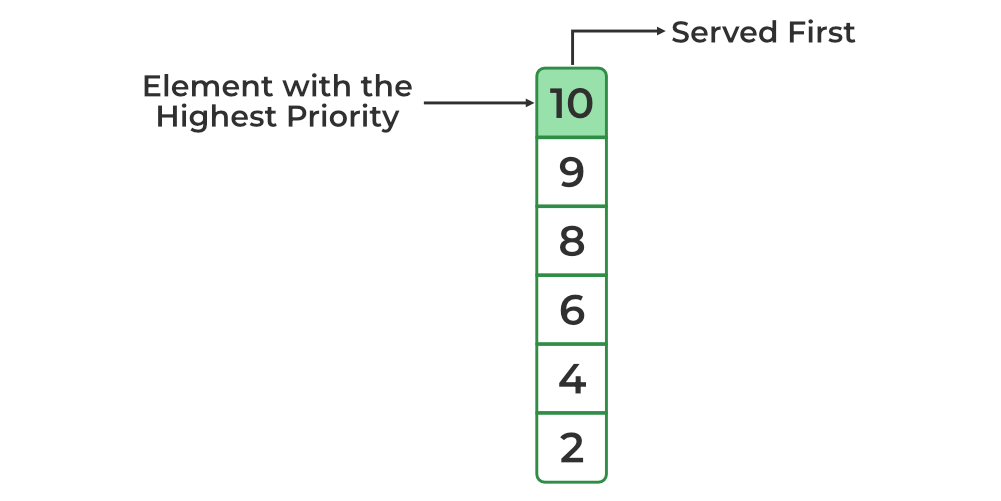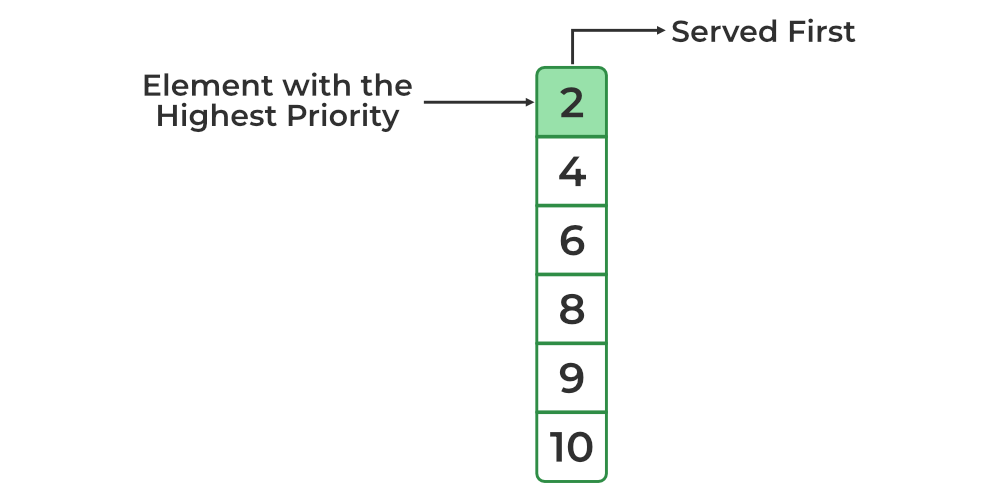GFG App
Open AppBrowser
Continue

# Priority Queue in C++ Standard Template Library (STL)

A C++ priority queue is a type of container adapter, specifically designed such that the first element of the queue is either the greatest or the smallest of all elements in the queue, and elements are in non-increasing or non-decreasing order (hence we can see that each element of the queue has a priority {fixed order}).

In C++ STL, the top element is always the greatest by default. We can also change it to the smallest element at the top. Priority queues are built on the top of the max heap and use an array or vector as an internal structure. In simple terms, STL Priority Queue is the implementation of Heap Data Structure.

Syntax:

`std::priority_queue<int> pq;`

Example:

## C++

 `// C++ program to demonstrate the use of priority_queue` `#include ` `#include ` `using` `namespace` `std;`   `// driver code` `int` `main()` `{` `    ``int` `arr = { 10, 2, 4, 8, 6, 9 };`   `    ``// defining priority queue` `    ``priority_queue<``int``> pq;`   `    ``// printing array` `    ``cout << ``"Array: "``;` `    ``for` `(``auto` `i : arr) {` `        ``cout << i << ``' '``;` `    ``}` `    ``cout << endl;` `    ``// pushing array sequentially one by one` `    ``for` `(``int` `i = 0; i < 6; i++) {` `        ``pq.push(arr[i]);` `    ``}`   `    ``// printing priority queue` `    ``cout << ``"Priority Queue: "``;` `    ``while` `(!pq.empty()) {` `        ``cout << pq.top() << ``' '``;` `        ``pq.pop();` `    ``}`   `    ``return` `0;` `}`

Output

```Array: 10 2 4 8 6 9
Priority Queue: 10 9 8 6 4 2 ```Max Heap Priority Queue (default scheme)

## How to create a min heap for the priority queue?

As we saw earlier, a priority queue is implemented as max heap by default in C++ but, it also provides us an option to change it to min heap by passing another parameter while creating a priority queue.

Syntax:

`priority_queue <int, vector<int>, greater<int>> gq;`

Example:

## C++

 `// C++ program to demonstrate` `// min heap for priority queue` `#include ` `#include ` `using` `namespace` `std;`   `void` `showpq(` `    ``priority_queue<``int``, vector<``int``>, greater<``int``> > gq)` `{` `    ``priority_queue<``int``, vector<``int``>, greater<``int``> > g = gq;` `    ``while` `(!g.empty()) {` `        ``cout << ``' '` `<< g.top();` `        ``g.pop();` `    ``}` `    ``cout << ``'\n'``;` `}`   `void` `showArray(``int``* arr, ``int` `n)` `{` `    ``for` `(``int` `i = 0; i < n; i++) {` `        ``cout << arr[i] << ``' '``;` `    ``}` `    ``cout << endl;` `}`   `// Driver Code` `int` `main()` `{` `    ``int` `arr = { 10, 2, 4, 8, 6, 9 };` `    ``priority_queue<``int``, vector<``int``>, greater<``int``> > gquiz(` `        ``arr, arr + 5);`   `    ``cout << ``"Array: "``;` `      ``showArray(arr, 6);`   `    ``cout << ``"Priority Queue : "``;` `    ``showpq(gquiz);`   `    ``return` `0;` `}`

Output

```The priority queue gquiz is :     2    4    6    8    9    10

gquiz.size() : 6
gquiz.top() : 2
gquiz.pop() :     4    6    8    9    10```Min Heap Priority Queue

Note: The above syntax may be difficult to remember, so in case of numeric values, we can multiply the values with -1 and use max heap to get the effect of min heap. Not only that we can use custom sorting method by replacing greater with custom comparator function.

## Methods of Priority Queue

Following list of all the methods of std::priority_queue class:

## Operations on Priority Queue in C++

### 1. Inserting and Removing Elements of a Priority Queue

The push() method is used to insert an element into the priority queue. To remove an element from the priority queue the pop() method is used because this removes the element with the highest priority.

Below is the C++ program for various functions in the priority queue:

## C++

 `// C++ Program to demonstrate various` `//  method/function in Priority Queue` `#include ` `#include ` `using` `namespace` `std;`   `// Implementation of priority queue` `void` `showpq(priority_queue<``int``> gq)` `{` `    ``priority_queue<``int``> g = gq;` `    ``while` `(!g.empty()) {` `        ``cout << ``' '` `<< g.top();` `        ``g.pop();` `    ``}` `    ``cout << ``'\n'``;` `}`   `// Driver Code` `int` `main()` `{` `    ``priority_queue<``int``> gquiz;` `    ``// used in inserting the element` `    ``gquiz.push(10);` `    ``gquiz.push(30);` `    ``gquiz.push(20);` `    ``gquiz.push(5);` `    ``gquiz.push(1);`   `    ``cout << ``"The priority queue gquiz is : "``;` `    `  `    ``// used for highlighting the element` `    ``showpq(gquiz);`   `    ``// used for identifying the size ` `    ``// of the priority queue` `    ``cout << ``"\ngquiz.size() : "` `<< ` `             ``gquiz.size();` `    ``// used for telling the top element ` `    ``// in priority queue` `    ``cout << ``"\ngquiz.top() : "` `<< ` `             ``gquiz.top();`   `    ``// used for popping the element ` `    ``// from a priority queue` `    ``cout << ``"\ngquiz.pop() : "``;` `    ``gquiz.pop();` `    ``showpq(gquiz);`   `    ``return` `0;` `}`

Output

```The priority queue gquiz is :  30 20 10 5 1

gquiz.size() : 5
gquiz.top() : 30
gquiz.pop() :  20 10 5 1```

Refer end for complexity analysis.

Note: Above shown is one of the methods of priority queue initialization. To know more about efficient initialization of priority queue, click here.

### 2. To Access the Top Element of the Priority Queue

The top element of the Priority Queue could be accessed using the top() method.

## C++

 `// C++ program to access the top` `// element of priority queue` `#include ` `#include ` `using` `namespace` `std;`   `// Driver code` `int` `main()` `{` `  ``// create a priority queue of int` `  ``priority_queue<``int``> numbers;`   `  ``// add items to priority_queue` `  ``numbers.push(1);` `  ``numbers.push(20);` `  ``numbers.push(7);`   `  ``// get the element at the top` `  ``cout << ``"Top element: "` `<< ` `           ``numbers.top();` `  ``return` `0;` `}`

Output

`Top element: 20`

Refer end for complexity analysis.

Note: We can only access the top element in the priority queue.

### 3. To Check whether the Priority Queue is Empty or Not:

We use the empty() method to check if the priority_queue is empty. This method returns:

• True – It is returned when the priority queue is empty and is represented by 1
• False – It is produced when the priority queue is not empty or False and is characterized by 0

Example:

## C++

 `// C++ program to demonstrate` `// Implementation of empty() function` `#include ` `#include ` `using` `namespace` `std;`   `// Driver code` `int` `main()` `{` `  ``priority_queue<``int``> pqueueGFG;` `  ``pqueueGFG.push(1);` `    `  `  ``// Priority Queue becomes 1` `  ``// check if it is empty or not` `  ``if` `(pqueueGFG.empty()) ` `  ``{` `    ``cout << ``"Empty or true"``;` `  ``}` `  ``else` `  ``{` `    ``cout << ``"Contains element or False"``;` `  ``}` `  ``return` `0;` `}`

Output

`Contains element or False`

Refer end for complexity analysis.

### 4. To Get/Check the Size of the Priority Queue

It determines the size of a priority queue. In simple terms, the size() method is used to get the number of elements present in the Priority Queue.

Below is the C++ program to check the size of the priority queue:

## C++

 `// C++ program to demonstrate the` `// size() method of priority queue` `#include ` `#include ` `using` `namespace` `std;`   `// Driver code` `int` `main()` `{` `  ``// create a priority queue of string` `  ``priority_queue pqueue;`   `  ``// add items to priority_queue` `  ``pqueue.push(``"Geeks"``);` `  ``pqueue.push(``"for"``);` `  ``pqueue.push(``"Geeks"``);` `  ``pqueue.push(``"C++"``);`   `  ``// get the size of queue` `  ``int` `size = pqueue.size();` `  ``cout << ``"Size of the queue: "` `<< size;`   `  ``return` `0;` `}`

Output

`Size of the queue: 4`

Refer end for complexity analysis.

### 5. To Swap Contents of a Priority Queue with Another of Similar Type

Swap() function is used to swap the contents of one priority queue with another priority queue of same type and same or different size.

Below is the C++ program to swap contents of a priority queue with another of similar type:

## C++

 `// CPP program to illustrate` `// Implementation of swap() function`   `#include ` `using` `namespace` `std;`   `// Print elements of priority queue` `void` `print(priority_queue<``int``> pq)` `{` `    ``while` `(!pq.empty()) {` `        ``cout << pq.top() << ``" "``;` `        ``pq.pop();` `    ``}` `    ``cout << endl;` `}`   `int` `main()` `{` `    ``// priority_queue container declaration` `    ``priority_queue<``int``> pq1;` `    ``priority_queue<``int``> pq2;`   `    ``// pushing elements into the 1st priority queue` `    ``pq1.push(1);` `    ``pq1.push(2);` `    ``pq1.push(3);` `    ``pq1.push(4);`   `    ``// pushing elements into the 2nd priority queue` `    ``pq2.push(3);` `    ``pq2.push(5);` `    ``pq2.push(7);` `    ``pq2.push(9);`   `    ``cout << ``"Before swapping:-"` `<< endl;` `    ``cout << ``"Priority Queue 1 = "``;` `    ``print(pq1);` `    ``cout << ``"Priority Queue 2 = "``;` `    ``print(pq2);`   `    ``// using swap() function to swap elements of priority` `    ``// queues` `    ``pq1.swap(pq2);`   `    ``cout << endl << ``"After swapping:-"` `<< endl;` `    ``cout << ``"Priority Queue 1 = "``;` `    ``print(pq1);` `    ``cout << ``"Priority Queue 2 = "``;` `    ``print(pq2);` `    ``return` `0;` `}`   `// This code is contributed by Susobhan Akhuli`

Output

```Before swapping:-
Priority Queue 1 = 4 3 2 1
Priority Queue 2 = 9 7 5 3

After swapping:-
Priority Queue 1 = 9 7 5 3
Priority Queue 2 = 4 3 2 1 ```

Refer end for complexity analysis.

### 5. To emplace a new element into the priority queue container

Emplace() function is used to insert a new element into the priority queue container, the new element is added to the priority queue according to its priority. It is similar to push operation. The difference is that emplace() operation saves unnecessary copy of the object.

Below is the C++ program to emplace a new element into the priority queue container:

## C++

 `// CPP program to illustrate` `// Implementation of emplace() function`   `#include ` `using` `namespace` `std;`   `int` `main()` `{` `    ``priority_queue<``int``> pq;` `    ``pq.emplace(1);` `    ``pq.emplace(2);` `    ``pq.emplace(3);` `    ``pq.emplace(4);` `    ``pq.emplace(5);` `    ``pq.emplace(6);` `    ``// Priority queue becomes 1, 2, 3, 4, 5, 6`   `    ``// Printing the priority queue` `    ``cout << ``"Priority Queue = "``;` `    ``while` `(!pq.empty()) {` `        ``cout << pq.top() << ``" "``;` `        ``pq.pop();` `    ``}`   `    ``return` `0;` `}`   `// This code is contributed by Susobhan Akhuli`

Output

`Priority Queue = 6 5 4 3 2 1 `

Refer end for complexity analysis.

### 6. To represent the type of object stored as an element in a priority_queue

The priority_queue :: value_type method is a built-in function in C++ STL which represents the type of object stored as an element in a priority_queue. It acts as a synonym for the template parameter.

Syntax:

`priority_queue::value_type variable_name`

Below is the C++ program to represent the type of object stored as an element in a priority_queue:

## C++

 `// C++ program to illustrate the` `// priority_queue :: value_type function`   `#include ` `using` `namespace` `std;`   `// Driver code` `int` `main()` `{` `    ``// declare integer value_type for priority queue` `    ``priority_queue<``int``>::value_type AnInt;`   `    ``// declare string value_type for priority queue` `    ``priority_queue::value_type AString;`   `    ``// Declares priority_queues` `    ``priority_queue<``int``> q1;` `    ``priority_queue q2;`   `    ``// Here AnInt acts as a variable of int data type` `    ``AnInt = 20;` `    ``cout << ``"The value_type is AnInt = "` `<< AnInt << endl;` `    ``q1.push(AnInt);` `    ``AnInt = 30;` `    ``q1.push(AnInt);` `    ``cout << ``"Top element of the integer priority_queue is: "` `         ``<< q1.top() << endl;`   `    ``// here AString acts as a variable of string data type` `    ``AString = ``"geek"``;` `    ``cout << endl` `         ``<< ``"The value_type is AString = "` `<< AString` `         ``<< endl;` `    ``q2.push(AString);` `    ``AString = ``"for"``;` `    ``q2.push(AString);` `    ``AString = ``"geeks"``;` `    ``q2.push(AString);`   `    ``cout << ``"Top element of the string priority_queue is: "` `         ``<< q2.top() << endl;`   `    ``return` `0;` `}`   `// This code is contributed by Susobhan Akhuli`

Output

```The value_type is AnInt = 20
Top element of the integer priority_queue is: 30

The value_type is AString = geek
Top element of the string priority_queue is: geeks```

Refer end for complexity analysis.

### Complexities Of All The Operations:

My Personal Notes arrow_drop_up